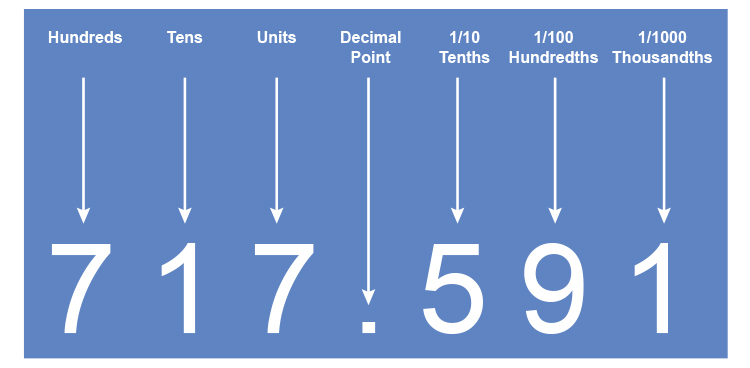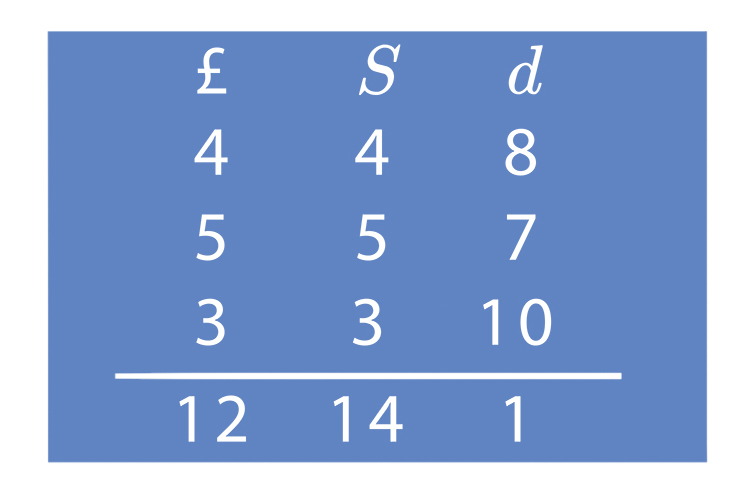Requires you to understand that the number such as 385 is in fact three 100’s eight 10’s and five 1’s.

Or           300 + 80 + 5

And this place value system is called the decimal system.Remember this is better than adding pounds shillings and pence.NOTE:

d = pennies = 12 pennies in a shilling

S = shillings = 20 shillings in a pound

£ = Pound = 240 pence (12xx20=240)

and 8d+7d+10d=25d or 2 shillings and 1d

and 4S+5S+3S and 2S carried over is 14 shillings

Example 1

398+427

 3 9 8 4 2 7 8 2 5

Answer: =825

To see this example step by step

Example 1

398+427

 3 9 8 + 4 2 7

 1 3 9 8 Add the ones (8 ones +7 ones =15 ones) + 4 2 7 Regroup (15 ones =1 ten +5 ones) 5

 1 1 3 9 8 Add the tens (1 ten +9 tens +2 tens =12 tens) + 4 2 7 Regroup (12 tens =1 hundred +2 tens) 2 5

 1 1 3 9 8 Add the hundreds (1 hundred +3 hundred +4 hundred =8 hundred) + 4 2 7 8 2 5

Answer: =825

Example 2

365+297

 3 6 5 2 9 7 6 6 2

Answer: =662

To see this example step by step

Example 2

365+297

 3 6 5 + 2 9 7

 1 3 6 5 Add the ones (5 ones +7 ones =12 ones) + 2 9 7 Regroup (12 ones =1 ten +2 ones) 2

 1 1 3 6 5 Add the tens (1 ten +6 tens +9 tens =16 tens) + 2 9 7 Regroup (16 tens =1 hundred +6 tens) 6 2

 1 1 3 6 5 Add the hundreds (1 hundred +3 hundred +2 hundred =6 hundred) + 2 9 7 6 6 2

Answer: =662